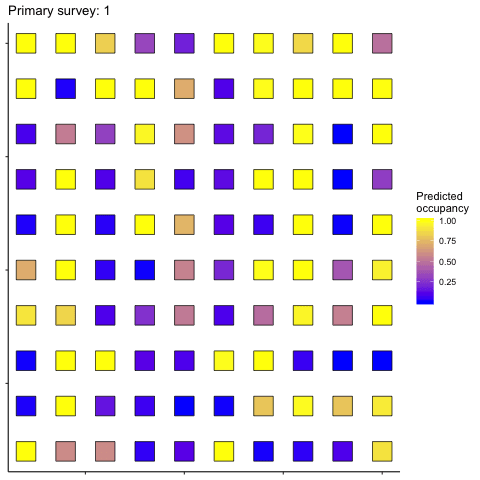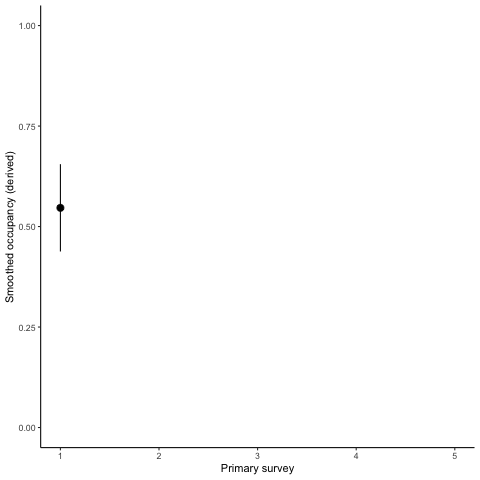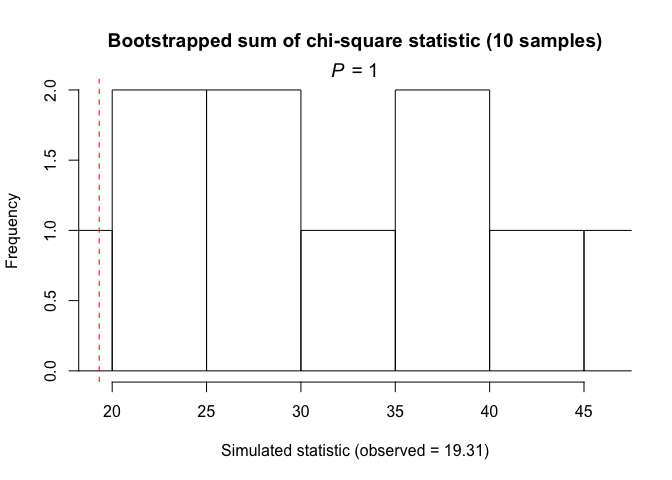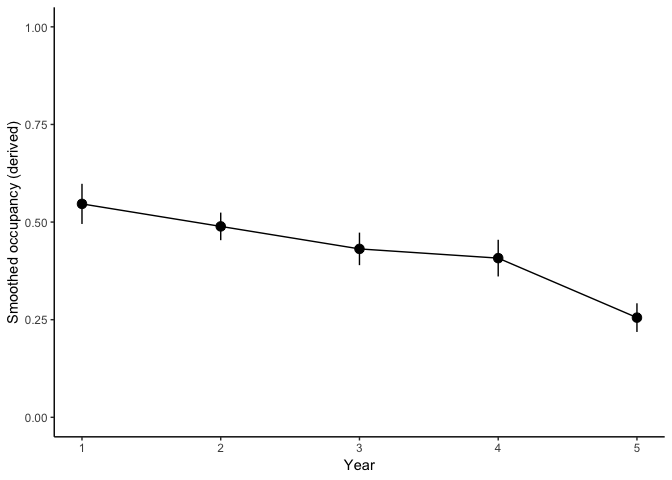In this tutorial, I cover:

1. The difference between single season (static) and multi-season (dynamic) occupancy models,
2. Fitting dynamic occupancy models with the R package unmarked, and
3. Making inferences, predictions, and plotting results from dynamic occupancy models.

The code (and sample data) from this tutorial are available on GitHub.

## Static versus Dynamic Occupancy Models

In the single-season (static) occupancy model, the occupancy state at any site does not change during the study. In other words, occupied sites stay occupied throughout the study. Conversely, unocuppied sites remain unoccupied throughout the study.

In reality, and over timeframes that are of interest to ecologists, occupied sites ‘blink-out’ due to extinctions and unoccupied sites become colonized. Dynamic occupancy models extend the single-season scenario by adding parameters to describe these processes. For the original and complete model description, read MacKenzie et al. 2003 (in reference list below).

The two added parameters:

• colonization probability ($$\gamma_i,_t$$) is the probability of an unoccupied site i becoming colonized between time t and t+1
• extinction probability ($$\epsilon_i,_t$$) is the probability of an occupied site i becoming extinct between time t and t+1

There are two other parameters, which are similar to the single-season occupancy models:

• detection probability ($$p_i,_t$$), the probability of finding a species at time t if it is present at site i)
• initial occupancy ($$\Psi_i$$), the probability that a species occurs at site i at the first survey (t = 1).

So, now we’re working with four parameter types, instead of two.

The other main difference is how we consider survey events. In single-season occupancy models, all surveys are equivalent in the sense that occupancy does not change between events. In dynamic occupancy models there are primary and secondary surveys (time is heirarchical). Occupancy status only changes between primary surveys, and secondary surveys are repeat visits to account for imperfect detection.

For example, we may assume that occupancy within a year does not change at a site (year = primary season). Within a year, we visit sites repeatedly to survey for a species (each visit within a year = secondary survey). The distinction between primary and secondary surveys depends on the biology of the study species, and on the experimental design for repeated visits.

Enough talk, let’s look at an example.

## Building dynamic occupancy models with unmarked

To get started, we need data describing on which surveys that a species was detected. We will use some sample data I simulated that represent surveys for frogs calling where we assume occupancy status does not change within a year. There are 3 surveys per year at each site over 5 years (15 total site visits).

The unmarked package uses a special object type to organize detection data and covariates: an unmarkedMultFrame for dynamic occupancy models.

library(dplyr) # for data organization
library(magrittr) # for piping
library(ggplot2) # for plotting
# install.packages("unmarked") # first time only
library(unmarked) # for occupancy models
library(AICcmodavg) # For GOF tests

# Load detection history (100 sites with 15 visits each)
detection_history <- read.csv("dynamic_detection_history.csv",
# First variable ("X") has row.names but not data
row.names = "X")

# Examine data
head(detection_history)
##   V1 V2 V3 V4 V5 V6 V7 V8 V9 V10 V11 V12 V13 V14 V15
## 1  1  0  0  0  0  0  0  0  0   0   0   0   0   0   0
## 2  0  0  0  0  0  0  1  0  1   0   0   0   0   0   0
## 3  0  0  0  0  0  0  0  0  0   0   0   0   0   0   0
## 4  0  0  0  0  0  0  0  0  1   0   0   0   0   0   0
## 5  0  0  0  0  0  0  0  0  0   0   1   0   0   0   0
## 6  0  1  0  1  0  1  0  0  0   0   0   0   0   0   0
# Load covariate data
effort <- read.csv("dynamic_effort.csv",
# First variable ("X") has row.names but not data
row.names = "X")
site_cov <- read.csv("dynamic_site_cov.csv",
# First variable ("X") has row.names but not data
row.names = "X")

# Build an unmarkedmultFramOccu
frog_unmarkedMultFrame <- unmarkedMultFrame( # y is a matrix with observed detection history
# 0's and 1's, one row per site, one column per survey
y = as.matrix(detection_history),
# numPrimary is the number of primary surveys;
# function derives # of secondary surveys based on
# ncol(y) / numPrimary
numPrimary = 5,
# obsCovs = observation covariates in a list,
# each variable has site rows x survey columns
obsCovs = list(effort = effort),
# siteCovs = dataframe with site rows x column variables
# the first column of site_cov is the site number
# the 5th:8th columns are used below in yearlySiteCovs
siteCovs = site_cov[,2:4],
# "yearlySiteCovs" are a list for site variables that differ between primary survey periods.
# In our example, the amount of wetland changes between primary survey periods and
# This data is stored within site_cov. There must be one column per primary survey (5 in our example)
yearlySiteCovs = list(wetland_time = site_cov[,c(2, 5:8)]))

# S4 class for colext occupancy model data
summary(frog_unmarkedMultFrame)
## unmarkedFrame Object
##
## 100 sites
## Maximum number of observations per site: 15
## Mean number of observations per site: 15
## Number of primary survey periods: 5
## Number of secondary survey periods: 3
## Sites with at least one detection: 57
##
## Tabulation of y observations:
##    0    1
## 1376  124
##
## Site-level covariates:
##     wetland            temp               prec
##  Min.   :0.1354   Min.   :-11.3766   Min.   :-10.1624
##  1st Qu.:0.3946   1st Qu.: -2.3297   1st Qu.: -2.7565
##  Median :0.4968   Median :  0.5792   Median :  0.3899
##  Mean   :0.4948   Mean   :  0.3977   Mean   :  0.3369
##  3rd Qu.:0.6074   3rd Qu.:  3.1949   3rd Qu.:  2.9048
##  Max.   :0.8839   Max.   : 12.1053   Max.   : 13.3464
##
## Observation-level covariates:
##      effort
##  Min.   :-17.2842
##  1st Qu.: -3.1384
##  Median :  0.2933
##  Mean   :  0.1404
##  3rd Qu.:  3.2423
##  Max.   : 17.7846
##
## Yearly-site-level covariates:
##   wetland_time
##  Min.   :0.006731
##  1st Qu.:0.259792
##  Median :0.360974
##  Mean   :0.377919
##  3rd Qu.:0.494461
##  Max.   :0.883868

Now that the data are organized, we can build models using the function colext(). We’ll start with a model where:

• initial occupancy ($$\Psi$$) is related to wetland amount (in the first year), temperature (temp), and precipitation (prec)
• detection probability ($$p$$) is related to search effort (scaled in our data to mean = 0, sd = 1)
• colonization probability ($$\gamma$$) is related to wetland amount (changes each year)
• extinction probability ($$\epsilon$$) is related to wetland amount (changes each year)

The variables for each parameter are specified with equations and must match the names within our unmarkedMultFrame (frog_unmarkedMultFrame).

dynamic_occ_m1 <- colext(
# Psi depends on initial habitat, climate
psiformula= ~wetland + temp + prec,
# colonization depends on wetland change
gammaformula = ~wetland_time,
# extinction depends on wetland change
epsilonformula = ~wetland_time,
# detection depends on survey effort
pformula = ~effort,
# data must be a unmarkedMultFrame
data = frog_unmarkedMultFrame,
# method is optim method, leave as "BFGS"
method = "BFGS")

Before we look at our results, we should check that the model fits the data reasonably well and that there is not strong evidence for assumption violations. A simple way to test this is to use Mackenzie-Bailey Goodness-of-Fit tests, which simulate detection data based on the specified model and compares fit to simulated fit with a Chi-square test (see previous tutorials in reference list for a more complete description).

Below, I include code to perform the GOF on our data, but I have limited nsim to 10 replicates, which takes my computer about one minute. The simulations are most useful with a much larger number (1000 to 5000). In our example today, we’re going to assume that based on a low number of simulations, the general model fits sufficiently. Remember that c-hat (variance inflation factors) should be ~1.

Extending the GOF to dynamic occupancy models involves simulating data for each “season” (here year) and calculating a Chi-square statistic. The function mb.gof.test() can handle dynamic models created with colext().

# Mackenzie-Bailey GOF test
# Simulate capture history data (if model correct). Compare obs X2 to sim X2
# Must simulate 1000-5000 times for good distribution estimates
# Likely to take a Very Long Time on large data sets
# with nsim = 10, takes my Mackbook ~ 60 seconds
mb.boot <- AICcmodavg::mb.gof.test(dynamic_occ_m1,
nsim = 10) # Must be much higher than five to be usefulprint(mb.boot, digit.vals = 4, digits.chisq = 4)
##
## Goodness-of-fit for dynamic occupancy model
##
## Number of seasons:  5
##
## Chi-square statistic:
## Season 1 Season 2 Season 3 Season 4 Season 5
##   3.5721   4.3276   3.5935   5.4571   2.3609
##
## Total chi-square = 19.3112
## Number of bootstrap samples = 10
## P-value = 1
##
## Quantiles of bootstrapped statistics:
##   0%  25%  50%  75% 100%
##   20   25   31   39   46
##
## Estimate of c-hat = 0.61

From our “too few” simulations, the model seems to fit okay and the c-hat estimate is less than one (possibly underdispersed). For now, we will assume this is not a problem.

Next, let’s look at the model output to try and better understand our system.

summary(dynamic_occ_m1)
##
## Call:
## colext(psiformula = ~wetland + temp + prec, gammaformula = ~wetland_time,
##     epsilonformula = ~wetland_time, pformula = ~effort, data = frog_unmarkedMultFrame,
##     method = "BFGS")
##
## Initial (logit-scale):
##             Estimate     SE      z P(>|z|)
## (Intercept)   0.6587 2.4982  0.264   0.792
## wetland      -1.1427 4.4104 -0.259   0.796
## temp          0.6440 0.3285  1.960   0.050
## prec         -0.0388 0.0903 -0.429   0.668
##
## Colonization (logit-scale):
##              Estimate    SE      z P(>|z|)
## (Intercept)     -2.19 0.947 -2.317  0.0205
## wetland_time     1.10 2.141  0.512  0.6085
##
## Extinction (logit-scale):
##              Estimate   SE     z P(>|z|)
## (Intercept)      2.02 1.18  1.71  0.0872
## wetland_time    -6.46 2.81 -2.30  0.0215
##
## Detection (logit-scale):
##             Estimate     SE     z  P(>|z|)
## (Intercept)  -1.4451 0.1631 -8.86 7.92e-19
## effort        0.0583 0.0215  2.72 6.62e-03
##
## AIC: 799.0299
## Number of sites: 100
## optim convergence code: 0
## optim iterations: 87
## Bootstrap iterations: 0

What does our model output from summary() indicate?

• Initial occupancy is not strongly related to the amount of wetland (estimate uncertain), is positively related to temperature (estimate positive), and is not strongly related to the amount of precipitation (estimate close to 0)
• Colonization is not strongly related to the amount of wetland (estimate close to 0)
• Extinction rate is negatively related to wetland (estimate negative)
• Detection probability increases with search effort

In previous tutorials on single-season occupancy models, I showed how we can build model subsets, compare models, and model-average. For this example, we will use just one model to predict occupancy at each site through time.

Remember that predicted occupancy probabilities (other than in the first primary survey) are derived using the parameters and are not directly estimated in the model. There are two types of derived occupancy probabilities the model output gives.

1. projected values represent population-level estimates
2. smoothed values represent finite-sample estimates

For more information on distinguishing these, see the vignette on colext and Weir et al. (2009) in the reference list below.

We are going to focus on smoothed values, to make inferences based just on our finite-samples. We may be interested in:

1. The predicted occupancy probability for each site during each primary survey (year). This is stored in an array within the model object (dynamic_occ_m1@smoothed). The array is 2 (a vector of probabilities for 1. unoccupied and 2. occupied) x 5 (number of primary surveys) x 100 (number of sites).
# Get the smoothed occupancy probabilities for just site 25 (at year 1:5)
# [2, , 25] = 2 for occupied, " " for all 5 surveys, 25 for site 25; remove [] to print whole array
dynamic_occ_m1@smoothed[2, , 25]
##  0.09276165 1.00000000 0.75861219 0.52574403 0.32415575

So, site 25 had an occupancy probability of 0.09 in year 1, 1.0 in year 2, 0.76 in year 3, etc.

1. The mean predicted occupancy among sites during each primary survey is also stored in the model object (dynamic_occ_m1@smoothed.mean). In other words, this is the predicted proportion of sampled sites that are occupied during each primary survey. The mean smoothed occupancy predictions are useful for looking at trends (i.e. is our frog species increasing or decreasing in occupancy?).
# Mean smoothed occupancy probabilities can be accessed using:
# dynamic_occ_m1@smoothed.mean, or
# smoothed(dynamic_occ_m1)

# Calculate SE for derived occupancy predictions using bootstrap, larger B requires longer time
m1 <- nonparboot(dynamic_occ_m1,
B = 10)

# Predicted occupancy in each year (with SE)
# the "[2,]" calls the occupied estimates,
# "[1,]" for unoccupied estimates
predicted_occupancy <- data.frame(year = c(1:5),
smoothed_occ = smoothed(dynamic_occ_m1)[2,],
SE = m1@smoothed.mean.bsse[2,])

Now we have an estimate (with uncertainty) of the proportion of study sites occupied during each year. Let’s plot it:Uh-oh, looks like our example frog species is declining in occupancy through our study. This was just an introduction, but we could test what variables affect colonization and extinction to better understand the dynamics of this system.

That’s all for now! We covered fitting dynamic (multi-season) occupancy models, testing goodness-of-fit, and predicting derived occupancy probabilities at each site and for the finite sample of surveyed sites.

Have suggestions for a tutorial you want on spatial ecology, mark-recapture models, occupancy models, or another related topic? Let me know!

## Further reading and references

1. Introduction to occupancy models in R

2. Occupancy models in R part 2: model comparisons

3. unmarked Vignette on colext https://cran.r-project.org/web/packages/unmarked/vignettes/colext.pdf

4. MacKenzie DI, Nichols JD, Hines JE, et al. 2003. Estimating site occupancy, colonization, and local extinction when a species is detected imperfectly. Ecology 84: 2200–2207.

5. Weir et al. 2009. Trends in anuran occupancy from northeastern states of the North American Amphibian Monitoring Program. Herpetological Conservation and Biology 4:389–402.